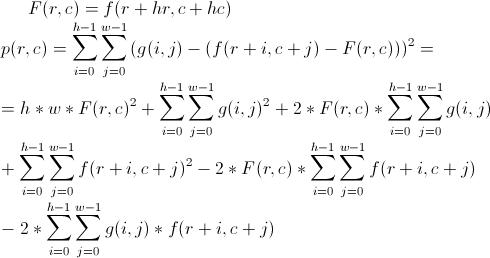HARD

### EXPLANATION

The straightforward approach to solving this problem would be to compute the score for every position (r,c). Unfortunately, a quick estimate shows that it won’t be fast enough. The problem looks similar to some kind of image filtering. If you’re familiar with that field or with the use of filters in digital signal processing, you could sense that it will have something to do with Fast Fourier Transform. Let’s begin by breaking down the score function which we’re trying to evaluate at every (r,c).

p(r,c) is the function which we are evaluating and (hr,hc) is the offset of the restaurant in chef’s layout. f(r,c) represents the height of the parcel and g(i,j) represents the relative height in preferred layout.First three terms are not problematic. We can precompute a table of cumulative sums and later compute the fourth and fifth term in O(1) as well. The hard part is taking care of the last/sixth term. It is in fact a cross-correlation, which can be formulated as convolution. So the problem reduces to efficiently precomputing a convolution of country layout and preferred layout. That’s where you need FFT to do it in O(n * log(n)), n=R * C. Once we have that, we can evaluate p(r,c) in constant time, find best solutions and output them.

However, FFT is hiding two problems. One of them are rounding errors related to floating-point computations. You needn’t worry about it, because the values in this problem are very small. The other problem is a relatively big constant, which is hiding in front of the n*log(n) time complexity. Implementing an efficient convolution using FFT is a challenge, so here are some ideas:

· if you are coding in C++, stay away from STL for this purpose. Header “complex” might be tempting, but it’s slow.
· recursive implementations tend to outperform iterative ones on personal computers because they make better use of CPU’s cache
· computing 2D FFT (first by rows and then by columns) proved to be faster for the same reason
· FFT of a real-valued sequence can be computed twice as fast as that of a complex-valued sequence.
A slightly easier version computes FFT of two real sequences by merging them into a complex sequence and later reconstructing the results.